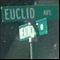Discussion about math, puzzles, games and fun.   Useful symbols: ÷ × ½ √ ∞ ≠ ≤ ≥ ≈ ⇒ ± ∈ Δ θ ∴ ∑ ∫  π  -¹ ² ³ °

You are not logged in.

## #1 2007-06-22 07:23:49

JaneFairfax
MemberRegistered: 2007-02-23
Posts: 6,868

### Binary relation

As Ive noted, a function can be formally defined as an ordered triple consisting of its domain, codomain, and graph: http://www.mathsisfun.com/forum/viewtop … 465#p74465. In fact, functions are special cases of the more general notion of binary relations.

A binary relation from X to Y is an ordered triple (X,Y,R), where R is a subset of X×Y. X, Y and R are called the domain, codomain and graph of the binary relation respectively  although if it is clear what X and Y are, it is much more usual to refer to the relation as just R rather than (X,Y,R). Also, for any xX, yY, if (x,y) ∈ R, it is much more usual to write xRy instead of (x,y) ∈ R.

Now consider the following four conditions which the binary relation R may satisfy:

(i) for any xX, there exists yY such that xRy
(ii) for any yY, there exists xX such that xRy
(iii) if xRy and x′Ry′, x = x′ ⇒ y = y
(iv) if xRy and x′Ry′, y = y′ ⇒ x = x

It can be seen that a function is a binary relation that satisfies (i) and (iii). If a binary relation satisfies (i), (ii) and (iii), it is a surjective function, while a binary relation that satisfies (i), (iii) and (iv) is an injective function. A bijection is a binary relation that satisfies all four conditions above.

Offline

## #2 2007-06-22 07:34:48

JaneFairfax
MemberRegistered: 2007-02-23
Posts: 6,868

### Re: Binary relation

Inverse binary relation

Let R be a binary relation from X to Y. The inverse of R, denoted R[sup]−1[/sup], is the binary relation from Y to X such that for any xX and yY, yR[sup]−1[/sup]x if and only if xRy.

It is obvious that

(a) R satisfies condition (i) above if and only if R[sup]−1[/sup] satisfies (ii)
(b) R satisfies (ii) if and only if R[sup]−1[/sup] satisfies (i)
(c) R satisfies (iii) if and only if R[sup]−1[/sup] satisfies (iv)
(d) R satisfies (iv) if and only if R[sup]−1[/sup] satisfies (iii)
(e) (R[sup]−1[/sup])[sup]−1[/sup] = R

In general, if  is a function, the inverse [sup]−1[/sup] is not a function, only a binary relation. [sup]−1[/sup] is a function if and only if  is bijective (in which case [sup]−1[/sup] itself is also bijective).

Last edited by JaneFairfax (2007-06-22 07:39:12)

Offline

## #3 2007-06-22 08:56:34

Ricky
ModeratorRegistered: 2005-12-04
Posts: 3,791

### Re: Binary relation

Something which I personally find fun is to try to invent sets and binary operators and then investigate to see if they have any cool properties.  Here is one I worked on a little, and always meant to get back to, but never have:

Rationals mod n.

Let a and b be integers, b non-zero.  Note that it need not be (a, b) = 1.  Define (a, b) + (c, d) as:

(ad + cb (mod n), bd (mod n) ) which would be the equivalent of:

And multiplication similarly.  See if you can find any properties such as abelian, commutative, identity, heck, even well defined.

"In the real world, this would be a problem.  But in mathematics, we can just define a place where this problem doesn't exist.  So we'll go ahead and do that now..."

Offline